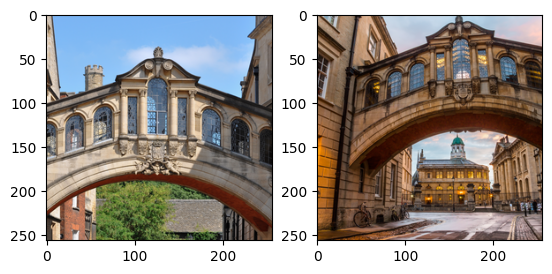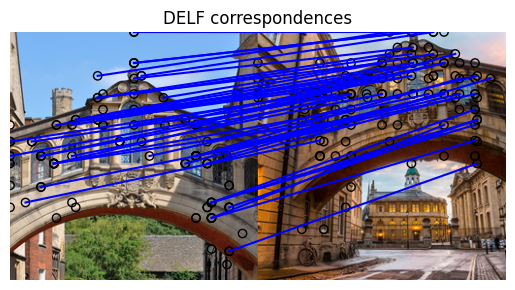Help protect the Great Barrier Reef with TensorFlow on Kaggle

How to match images using DELF and TensorFlow Hub

TensorFlow Hub (TF-Hub) is a platform to share machine learning expertise packaged in reusable resources, notably pre-trained modules.

In this colab, we will use a module that packages the DELF neural network and logic for processing images to identify keypoints and their descriptors. The weights of the neural network were trained on images of landmarks as described in this paper.

Setup

pip install scikit-image
from absl import logging

import matplotlib.pyplot as plt
import numpy as np
from PIL import Image, ImageOps
from scipy.spatial import cKDTree
from skimage.feature import plot_matches
from skimage.measure import ransac
from skimage.transform import AffineTransform
from six import BytesIO

import tensorflow as tf

import tensorflow_hub as hub
from six.moves.urllib.request import urlopen

The data

In the next cell, we specify the URLs of two images we would like to process with DELF in order to match and compare them.

Choose images

path = tf.keras.utils.get_file(url.split('/')[-1], url)
image = Image.open(path)
image = ImageOps.fit(image, (new_width, new_height), Image.ANTIALIAS)
return image

plt.subplot(1,2,1)
plt.imshow(image1)
plt.subplot(1,2,2)
plt.imshow(image2)
7020544/7013850 [==============================] - 0s 0us/step
7028736/7013850 [==============================] - 0s 0us/step
14172160/14164194 [==============================] - 1s 0us/step
14180352/14164194 [==============================] - 1s 0us/step
<matplotlib.image.AxesImage at 0x7f333b5e2d10>Apply the DELF module to the data

The DELF module takes an image as input and will describe noteworthy points with vectors. The following cell contains the core of this colab's logic.

def run_delf(image):
np_image = np.array(image)
float_image = tf.image.convert_image_dtype(np_image, tf.float32)

return delf(
image=float_image,
score_threshold=tf.constant(100.0),
image_scales=tf.constant([0.25, 0.3536, 0.5, 0.7071, 1.0, 1.4142, 2.0]),
max_feature_num=tf.constant(1000))
result1 = run_delf(image1)
result2 = run_delf(image2)

Use the locations and description vectors to match the images

TensorFlow is not needed for this post-processing and visualization

def match_images(image1, image2, result1, result2):
distance_threshold = 0.8

num_features_1 = result1['locations'].shape
print("Loaded image 1's %d features" % num_features_1)

num_features_2 = result2['locations'].shape
print("Loaded image 2's %d features" % num_features_2)

# Find nearest-neighbor matches using a KD tree.
d1_tree = cKDTree(result1['descriptors'])
_, indices = d1_tree.query(
result2['descriptors'],
distance_upper_bound=distance_threshold)

# Select feature locations for putative matches.
locations_2_to_use = np.array([
result2['locations'][i,]
for i in range(num_features_2)
if indices[i] != num_features_1
])
locations_1_to_use = np.array([
result1['locations'][indices[i],]
for i in range(num_features_2)
if indices[i] != num_features_1
])

# Perform geometric verification using RANSAC.
_, inliers = ransac(
(locations_1_to_use, locations_2_to_use),
AffineTransform,
min_samples=3,
residual_threshold=20,
max_trials=1000)

print('Found %d inliers' % sum(inliers))

# Visualize correspondences.
_, ax = plt.subplots()
inlier_idxs = np.nonzero(inliers)
plot_matches(
ax,
image1,
image2,
locations_1_to_use,
locations_2_to_use,
np.column_stack((inlier_idxs, inlier_idxs)),
matches_color='b')
ax.axis('off')
ax.set_title('DELF correspondences')
match_images(image1, image2, result1, result2)